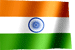RAMESH KRISHNA REDDYOnline Tutorials | PREV | TOP | NEXT

DRONA SERIES
COBOL STUDY MATERIAL

COBOL VERBS - COMPUTE

COBOL VERBS - COMPUTE

COMPUTE Verb All the arthemetic, that we can done using verb ADD, SUBTRACT, MULTIPLY, DIVIDE verbs can be done using COMPUTE statement. In COMPUTE statement, we need to use following operators to do arthemetic.
 operator Meaning + Add - Subtract ** Exponentiation / Divide * Multiplication
Format. COMPUTE < data-item-1> [ROUNDED] ... = arithmetic-expression [ ON SIZE ERROR < imperative statement-1 ] .. [ NOT ON SIZE ERROR < imperative-statement-2> ] .. Example 1 - COMPUTE WS-A = WS-B + WS-C - WS-D. Values in WS-B , WS-C will be added , WS-D value subtracted from that value and store the final result in WS-A. data-items values values before exectuion after execution WS-A 300 850 <--- changed WS-B 800 800 WS-C 100 100 WS-D 050 050 It is very important to know the order of evaluation, i.e.., In which order operations are performed in COMPUT statement. Below table , explains us, the order of evalution. Priority 1 ** Priority 2 * or / ( If both appeared , first appeared operator executed first, from left to right ) Priority 3 + or - ( If both appeared , first appeared operator executed first, from left to right ) If parentheses appear in the COMPUTE statement, it will override above sequence priority. Operations within parentheses are performed first. Statement Order of evaluation WS-A + 10 ** 2 Exponentiation ( 10 ** 2) executed first and the result 100 will be added to WS-A (WS-A + 10 ) ** 2 Addition executed first and exponentiation follows. ROUNDED - This option is available with all arithmetic VERBs and it is optional. COMPUTE WS-A = 23.456 + 20.034 When we sum values 23.456 and 20.034 we get the result 40.490. But in this case WS-A picture clause is 99v9 means it can store only 1 decimal position, after executing above statement WS-A contains the value of 40.4 value 90 will get trun- cated. More desirable value is 40.5 in this case. rounded to nearest value. This can be acheived thru the use of ROUNDED option. COMPUTE WS-A ROUNDED = 23.456 + 20.034 after execution of above statement WS-A contains value of 40.5 ON SIZE ERROR Let take some example to understand this option. 01 WS-A PIC 9(3) VALUE 400. 01 WS-B PIC 9(3) VALUE 800. 01 WS-C PIC 9(3) VALUE 300. COMPUTE WS-A = WS-B + WS-C. Above statement, add the value in WS-B and WS-C and store that value in WS-A. After execution of above statement WS-A contains the value of 100, instead of 1100. Because WS-A can hold only upto 3 bytes. Please note that, program wont get abended because this overflow/truncation condition. But value moved to WS-A is not correct one. To aviod these kind of size errors, best procedure is make sure receiving field has defined with large enough size to accommodate the result. But sometimes, programmer may not know the input max number/forget to define the receiving fields with enough sizes. It is a good practice to use ON SIZE ERROR to catch such errors. ON SIZE ERROR option can be used with following arithmetic statements. - ADD ... ON SIZE ERROR ... . - SUBTRACT ... ON SIZE ERROR ... . - MULTIPLY ... ON SIZE ERROR ... . - DIVIDE ... ON SIZE ERROR ... . - COMPUTE ... ON SIZE ERROR ... . COMPUTE WS-A = WS-B + WS-C ON SIZE ERROR MOVE ZEROES TO WS-A. In above example, If WS-A cannot accomodate the result then ZEROES will be moved to WS-A. A size error can occurs in the following ways. - When receiving field is not large enough to accommodate the result. - When division by zero occurs If ON SIZE ERROR option becomes true, statements after this option will get executed. These statement needs to be ended either by period OR scope terminator i.e, in case of ADD, scope terminator is END-ADD. TIP : Always make sure receiving field has large enough to accommodate the result.

NEXT CHAPTER TOPIC : COBOL

Visit COBOL books section in this site for good books